## Friday, May 31, 2019

### THALES Salamandre HFXL bursts

In the last days THALES Salamandre HFXL signals were again active, as was also noticed by Antonio.

TDoA points to the area around Paris. However, judging from the observed field strengths, there were transmitters at (at least) two locations active.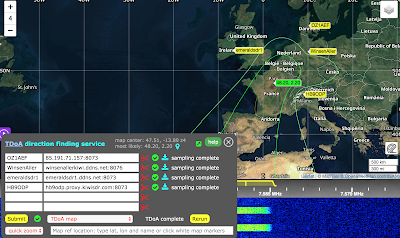TDoA

A 72-frame long HFXL burst is shown below (most bursts consist of 9 frames). The symbols were obtained by manually correcting for the doppler offset and searching for the correct symbol timing using GNU octave. The burst consists of frames with 287 symbols where the last 31 symbols are +-sign miniprobes.HFXL burst

The preamble consists of three parts where the 1st two parts are following MIL-STD-188-110 App. C and the 3rd part is a proprietary extension. It encodes 10 di-bits D0,...,D9 containing information about the used modulation schema and other signal parameters.HFXL preamble

It is known that the first few di-bits encode information about the used modulation schema:
[D0, D1, D2] = [(1,M0), (1,M1), (1,M2)]
In order to study these bursts more systematically the extended HFXL preamble was implemented in gr-digitalhf as an extension of the 110C mode. A number of recorded HFXL signals were analyzed and the constellations shown below were found (not shown: BPSK). Note that the BPSK and QPSK modes are scrambled to 8PSK symbols.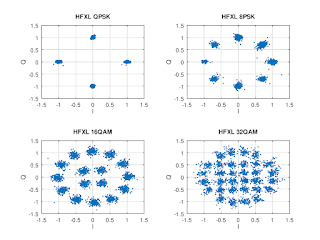HFXL constellations after descrambling

The table below shows a map between [M0,M1,M2] and the channel symbols after descrambling. 64QAM mode was not observed and is therefore missing in the table below.
 M0,M1,M2  Channel symbols after descrambling
0, 0, 0   BPSK
1, 0, 0   QPSK
0, 1, 0   8PSK
1, 1, 0  16QAM
0, 0, 1  32QAM
1, 0, 1   ----
0, 1, 1   QPSK chips with phases [+1,-1,-1,+1]
1, 1, 1   ----

At first is was puzzling that two combinations of [M0,M1,M2] correspond to QPSK descrambled symbols. Having a closer look at (0,1,1) bursts, it can be seen that it consists of QPSK symbols Si spread out using [+1,-1,-1,+1] chips, i.e.,
..., Si,-Si,-Si,Si, Si+1,-Si+1,-Si+1,Si+1, ...
making it a very robust mode to transmit data.QPSK (0,1,1) mode

The bits extracted from the 72-frame long burst shown above have a mean of 0.377; assuming that the probability for a bit to be 1 is 0.5 this translates into 24.6% of the payload consisting of zeros. If this is correct then one should see long strings of 0 bits.

Assuming that the 72 frames in this burst consist of two blocks of 36 frames, and that an interleaver of the form specified in MIL-STD-188-110 App. D is used, the maximum run lengths for all interleaver increments are shown below. For both interleaver blocks there is a maximum for an interleaver increment of 469.maximum run length as a function of interleaver increment

Assuming that the correct interleaver increment is 469, the deinterleaved bits are shown below:
• there are four gaps, each about 215 bits long
• the probability of a bit to be 1 if it is not in one of these gaps is 46.1% for the 1st block and 46.9% for the 2nd block
• the distribution of the deinterleaved bits for both blocks is similar and shows an interesting patterndeinterleaved bits for interleaver increment 469

As this analysis is based on a single burst with 72 frames, it might be a mere coincidence. In addition it is worth noticing that using the same interleaver increment, assuming that all 72 frames are a single interleaver block,  the double of the bit patterns above is obtained.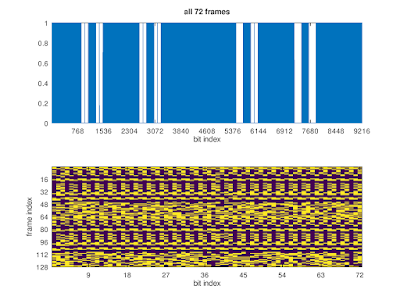deinterleaved bits for interleaver increment 469

## Wednesday, May 29, 2019

### MIL-STD-188-110 App.C QAM

Recently MIL-STD-188-110 App C  (STANAG 4539) signals were found on a KiwiSDR. This made it possible to complete the corresponding decoder in gr-digitalhf which includes an adaptive channel filter, descrambling, deinterleaving, and convolutional decoding. For the QAM modes descrambling is done by applying an XOR operation on the soft decisions.QAM32 constellation obtained after decoding with gr-digitalhf
Using the path metrics of the convolutional decoder it was verified that the whole chain of decoding steps is correct: without puncturing the path metric $$M$$ for $$n$$ encoded bits at rate $$1/2$$ is $$2n$$ if there are no errors, so it makes sense to use $$Q=M/2n$$ as a measure of data quality. When there is puncturing, e.g., to rate $$3/4$$ with puncturing pattern [11, 10], the expected path metric is $$3.5/4$$: three out of four bits are transmitted and the probability for the puncture to be correct is $$1/2$$. Therefore in this case $$Q$$ has to be rescaled by the factor $$4/3.5=8/7$$.

## Friday, May 10, 2019

### Blind detection of convolutional codes

This post continues the topic started in this blog post, i.e., the detection of the presence of convolutional coding in a given bit stream.

The method outlined below is not new and a good reference it is this paper: M. Marazin, R. Gautier, G. Burel “Blind recovery of k/n rate convolutional encoders in a noisy environment

The basic idea is to arrange a given bit stream $$b_i$$ in matrices $$R_k$$ \begin{equation} B_k = \begin{pmatrix} b_0 & b_1 & b_2 & \cdots & b_{k-1}\\ b_k & b_{k+1} & b_{k+2} & \cdots & b_{2k-1}\\ \vdots & \vdots & \vdots & \ddots & \vdots \end{pmatrix} \end{equation} and then to determine the rank of $$R_k = \mathsf{rank}(B_k)$$ as a function of $$k$$. Note that for computing the rank $$B_k$$ is treated as a matrix with values in $$\mathbb{F}_2$$.

If the bit stream is completely random, all matrices $$B_k$$ will likely have rank $$R_k=k$$. However, the redundancy introduced by convolutional coding shows up as some matrices not having full rank, i.e., there are dependent rows in some $$B_k$$ and therefore $$R_k < k$$ for some $$k$$.

Starting from a $$k=7,\;r=1/2$$ mother code, rank deficiency plots are shown below for different puncturing patterns, together with the expected behavior.

Note that this method detects any redundancy (error coding) in a given bit stream and therefore can be generalized to other coding schemes.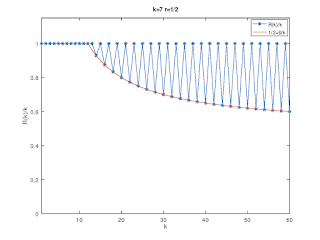Rank deficiencies for the k=7 r=1/2 mother code.Rank deficiencies for a k=7 r=2/3 code obtained by puncturing.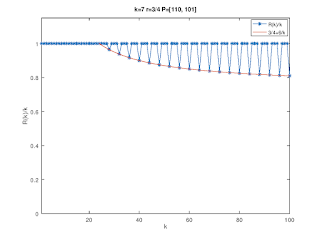Rank deficiencies for a k=7 r=3/4 code obtained by puncturing.Rank deficiencies for a k=7 r=4/5 code obtained by puncturing.

### MIL-STD-188-110D mini-probe base sequences

The mini-probes specified in MIL-STD-188-110D, Appendix D have interesting structures. Let us start with the two exceptions, $$n = 13$$ and $$n = 19$$:

### $$n = 13$$: (TABLE D-XXII)

This is the well-known Barker-13 sequence, i.e., \begin{equation} [+1, +1, +1, +1, +1, −1, −1, +1, +1, −1, +1, −1, +1]\;. \end{equation}

### $$n = 19$$: (TABLE D-XXIV)

This sequence is based on Legendre-19: \begin{equation} [1,\, -\mathsf{Legendre}(k/19)]\;. \end{equation}

### $$n = m^2$$: (TABLES D-XXIII, D-XXV - D-XXXVI)

All other mini-probe base sequences have lengths $$n=m^2$$ for $$4\le m \le 17$$ and can be obtained from the following formula, \begin{equation} MP(k;m) = \exp\left\{-2\pi i m \left\lfloor{\frac{k}{m}}\right\rfloor \frac{k}{n} \right\}\;, \end{equation} where $$\left\lfloor{x}\right \rfloor$$ denotes floor function which returns the greatest integer $$\leq x$$. Curiously, the mini-probe base sequences for $$m\ge 14$$ are the complex conjugate of the formula above, so I wonder if this is by design or it is an error in the standard.

Note that $$MP(k;4)$$ is the (complex conjugate of the) length-16 Frank-Heimiller sequence contained in TABLE C-VIII, i.e., \begin{equation} \exp\big\{2\pi i\, [0, 0, 0, 0,\; 0, 2, 4, 6,\; 0, 4, 0, 4,\; 0, 6, 4, 2]/8\big\}\;. \end{equation} Many pages could have been saved by describing the mini-probe base sequences in terms of the formula above.